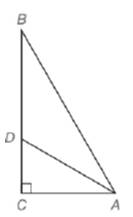Chapter 5.6, Problem 20E### Elementary Geometry for College St...

6th Edition
Daniel C. Alexander + 1 other
ISBN: 9781285195698

#### Solutions

Chapter
Section### Elementary Geometry for College St...

6th Edition
Daniel C. Alexander + 1 other
ISBN: 9781285195698
Textbook Problem
1 views

# Given: In Δ A B C , A D → bisects ∠ B A C A B = 20 and A C = 16Find: D C and D B

To determine

To find:

DC and DB.

Explanation

Given:

In ΔABC, AD bisects BAC, AB=20,AC=16.

Theorem used:

Angle bisector theorem:

If a ray bisects one angle of a triangle, then it divides the opposite side into segments whose lengths are proportional to the lengths of the two sides that form the bisected angle.

Calculation:

Then by angle-bisector theorem, the ray AD divides the opposite side into segments whose lengths are proportional to the lengths of the two sides that form the bisected angle.

ABAC=BDDC.

Since ABC is a right triangle, by Pythagoras theorem we get

(AB)2=(AC)2+(BC)2

We have AB=20,AC=16.

(AB)2=(AC)2+(BC)2(20)2=(16)2+(BC)2400256=(BC)2144=(BC)212=BC

We have ABAC=BDDC

### Still sussing out bartleby?

Check out a sample textbook solution.

See a sample solution

#### The Solution to Your Study Problems

Bartleby provides explanations to thousands of textbook problems written by our experts, many with advanced degrees!

Get Started

#### Find more solutions based on key concepts